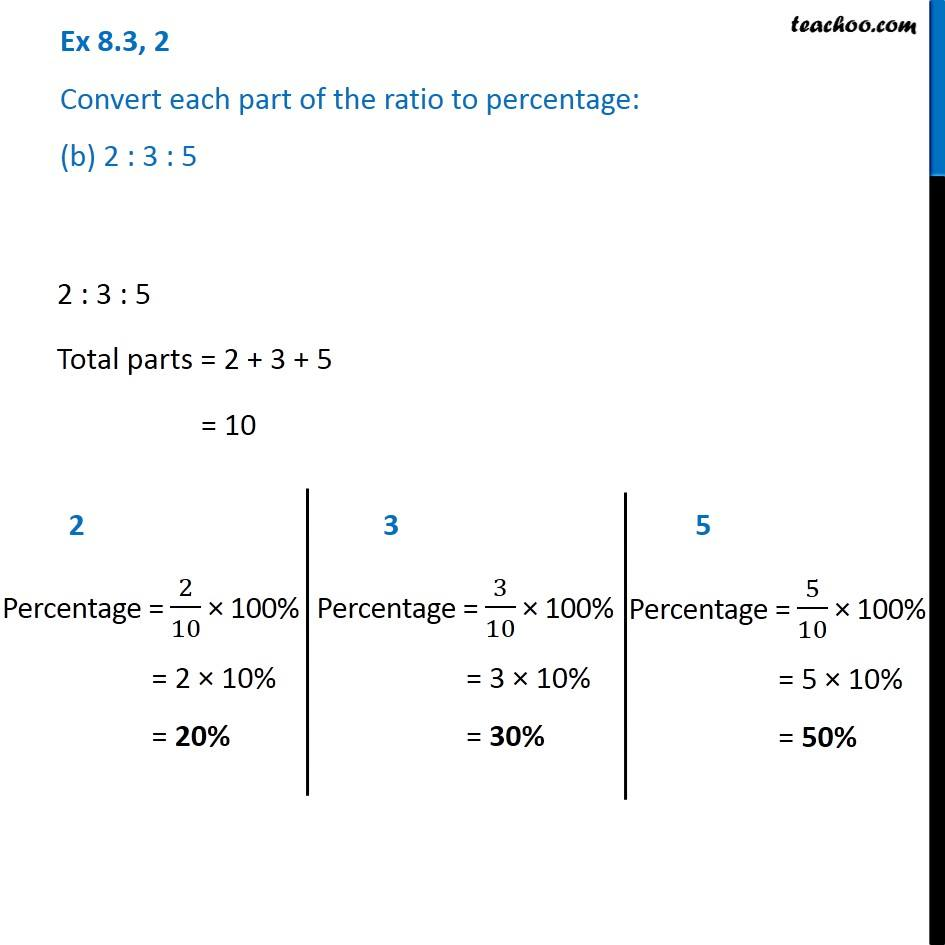Ex 8.3

Chapter 8 Class 7 Comparing Quantities
Serial order wiseGet live Maths 1-on-1 Classs - Class 6 to 12

### Transcript

Ex 8.3, 2 Convert each part of the ratio to percentage: (b) 2 : 3 : 5 2 : 3 : 5 Total parts = 2 + 3 + 5 = 10 2 Percentage = 2/10 × 100% = 2 × 10% = 20% 3 Percentage = 3/10 × 100% = 3 × 10% = 30% 5 Percentage = 5/10 × 100% = 5 × 10% = 50%